# Numbers to Text

In Excel, numbers are right-aligned and text is left-aligned. This example teaches you how to convert numbers to 'text strings that represent numbers'.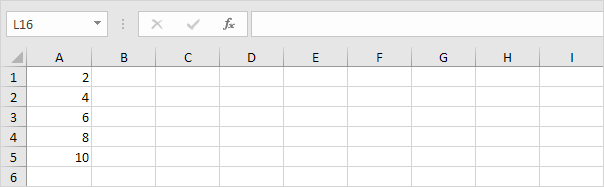1. Select the range A1:A4 and change the number format to Text.2. Precede a number by an apostrophe and it will also be treated as text.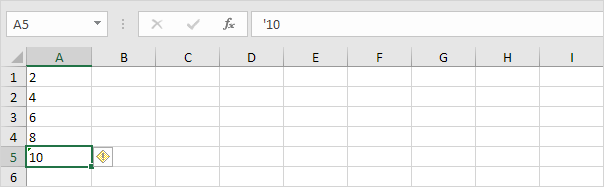3a. If you add text to a number and still want to format this number, use the TEXT function. Without using the TEXT function this would be the result.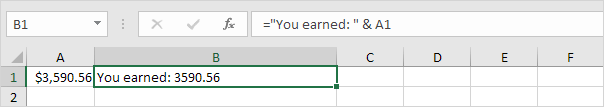3b. With the TEXT function.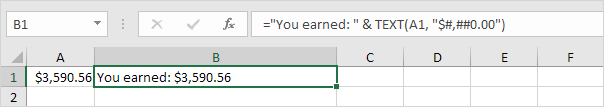Note: #,## is used to add comma's to large numbers.

3c. Here's another example. Apply a percentage format.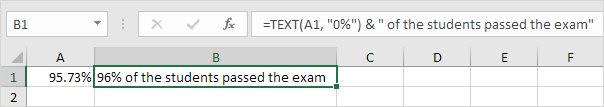Note: use 0 to display the nearest integer value. Use 0.0 for one decimal place. Use 0.00 for two decimal places, etc.

3d. And another example. Format a date.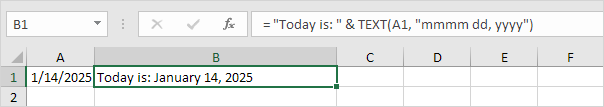Note: use less/more m's, d's and y's to change the appearance of the date. Visit our page about the TEXT function for many more examples.

Go to Next Chapter: Find & Select